# Survey of Some Topics Covered in CO 739¶

We give some examples of the topics covered in the course Analytic and Algorithmic Combinatorics at Waterloo in Winter 2021. To run the code in a cell, click on the cell then hit Shift+Enter. Feel free to play around and modify the code! You should run the code in each section from the beginning of the section to reduce errors.

## 1. Simple Random Walks in a Quadrant¶

In :
################################################################################################
# The following functions are used to generate animations of random walks in the first quadrant
################################################################################################
# Generate endpoints of random walk using steps in Steps
def walkSum(Steps):
wk = [[0, 0]]
for k in Steps:
wk.append([wk[-1]+k,wk[-1]+k])
return wk

# Find index walk wk leaves non-negative quadrant
def leaveIndex(wk):
for k in range(len(wk)):
if (wk[k]<0) or (wk[k]<0):
return k;
return len(wk)+1;

# Plot the state of the random walk wk at time k
# bd denotes the plot boundaries
def singlePlot(wk, k, bd):
if k < leaveIndex(wk):
oldSteps = line(wk[0:k], color='black', thickness=2)
newSteps = line(wk[k-1:k+1], color='red', thickness=2)
else:
oldSteps = line(wk[0:k], alpha=0.03, color='gray')
newSteps = line(wk[k-1:k+1], alpha=0.03, color='gray', thickness=2)
return oldSteps + newSteps

# Plot the state of all random walks in WK at time k
# bd denotes the plot boundaries
def singleListPlot(WK, k, bd):
plt = line([])
for wk in WK:
plt += singlePlot(wk,k,bd)

plt.set_axes_range(xmin=-bd,xmax=bd, ymin=-bd,ymax=bd)
numLeft = len(list(filter(lambda x:leaveIndex(x)>k,WK)))
txt = text("Number of steps: %s" %(k),(0,1.05),axis_coords=True, horizontal_alignment="left")
txt += text("Walks in first quadrant: %s" %(numLeft),(0,1),axis_coords=True,horizontal_alignment="left")

# Add plots and text, and set boundaries and aspect ratio
plt = plt + txt
plt.set_axes_range(xmin=-bd,xmax=bd, ymin=-bd,ymax=bd)
return plt

# Generate sequence of plots for first N steps of the random walks in WK
# bd denotes the plot boundaries
def plotWalk(WK, N, bd):
return [singleListPlot(WK,k,bd) for k in range(1,N+1)]

In :
# Define a set of steps
ST = [[1,0],[-1,0],[0,1],[0,-1]]

# Define the length of the walks (N) and the number of walks (K)
N = 300
K = 300

# Generate steps for K random walks of length N
StepSeq = [[choice(ST) for k in range(N)] for j in range(K)]

# Generate the random walks and find maximum coordinate
WK = [walkSum(Steps) for Steps in StepSeq]
bd = max(flatten(WK))

# Get sequence of plots and convert to Sage Animation class
ani = animate(plotWalk(WK, N, bd+1))

# Display the final frame of the animation
ani[-1]

Out: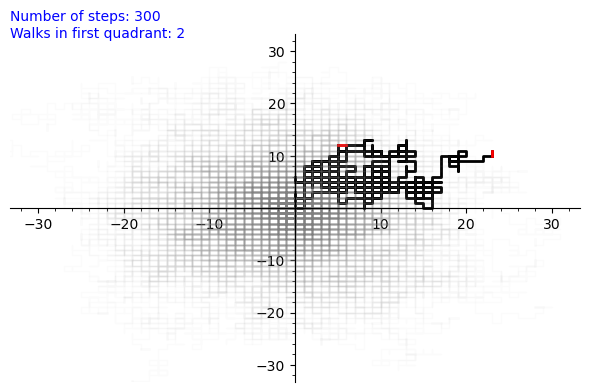In :
# Output and save the animation -- this *requires* extra software such as ImageMagick or ffmpeg
# Uncomment and run the following line to save the animation
# ani.save("WalkAnimation.mp4")


## 2. Complexity of Computing Virahanka-Fibonacci Numbers¶

The Virahanka-Fibonacci Numbers satisfy the recurrence relation $f_{n+2} = f_{n+1} + f_n$ for all $n \geq 0$, together with the initial conditions $f_0=f_1=1$.

#### Naive Function to Compute Terms¶

In :
# The following recursive function directly encodes the recurrence
# Note: '@cached_function' saves previously returned function values
# in memory, otherwise even computing the 100th term takes a *long* time
@cached_function
def fib(n):
if n==0 or n==1:
return 1;
else:
return fib(n-1) + fib(n-2)

print([fib(k) for k in range(10)])

[1, 1, 2, 3, 5, 8, 13, 21, 34, 55]

In :
# This is quite inefficient -- roughly, to compute the Nth term takes N^2*log(N) operations
# Note: running this cell more than once will give incorrect timings because of '@cached_function'
# Note: trying to compute more than the 1000th term will cause Sage to exceed its recursion depth
timeit('fib(10^2)')

Out:
625 loops, best of 3: 488 ns per loop

#### Binary Powering of a Matrix¶

In :
# The Fibonacci recurrence can be encoded as a matrix-vector product
M = Matrix(ZZ,2,2,[1,1,1,0])
v = Matrix(ZZ,2,1,[1,1])
print([(M^k * v)[1,0] for k in range(10)])

[1, 1, 2, 3, 5, 8, 13, 21, 34, 55]

In :
# To compute the Nth sequence term one can thus compute the Nth power of M
# This can be done efficiently using 'binary powering'
# Note: Sage has some built-in optimizations for matrix powers but we use this function to be explicit
def bin_pow(x,n):
if n<=0:
raise TypeError('Enter a positive integer')
elif n==1:
return x
# When n is even
elif n%2 == 0:
return bin_pow(x,n/2)^2
# When n is odd
else:
return bin_pow(x,(n-1)/2)^2*x

In :
# Matrix binary powering is much more efficient than the naive approach
timeit('bin_pow(M,10^2)')

Out:
625 loops, best of 3: 27.5 μs per loop
In :
# Here is the millionth Virahanka-Fibonacci Number
# It has 208,988 digits, so we print an approximation (remove '.n()' to see the exact value)
(bin_pow(M,10^6) * v)[1,0].n()

Out:
3.16047687386690e208987

#### Polynomial Multiplication in a Quotient Ring¶

In :
# The Fibonacci recurrence can also be encoded by a polynomial product
# First, define the quotient ring K = Q[x]/(x^2-x-1)
R.<x> = QQ['x']
J = R.ideal(x^2-x-1)
K = R.quo(J)

# Make xb the image of x in the residue ring K
xb = K(x)

# The nth Fibonacci number is the sum of the coefficients in xb^n
print([(xb^k).lift().subs(x=1) for k in range(10)])

[1, 1, 2, 3, 5, 8, 13, 21, 34, 55]

In :
timeit('bin_pow(M,10^2)')

Out:
625 loops, best of 3: 26.9 μs per loop
In :
# Using our refined approachs takes roughly N*log^2(N) operations to compute f_N.
# First, a helper function to parse the output of Sage's timeit command
def format_time(t):
a = t.stats
if a[-1] == 's':
return 10^6 * a[-2]
elif a[-1] == 'ms':
return 10^3 * a[-2]
elif a[-1] == 'μs':
return a[-2]
elif a[-1] == 'ns':
return 10^(-3) * a[-2]

# Now we time our methods on computing f_n for n = k*10^5 with k = 1,...,7
# Then plot the results (the linear complexity can be seen in the plots)
timesM = [timeit('bin_pow(M,' + str(k) + '*10^5)') for k in range(1,8)]
ptM = point([(k*10^5,format_time(timesM[k-1])) for k in range(1,8)], color='red', size=30, axes_labels=['$N$', 'μs'])
timesQ = [timeit('bin_pow(xb,' + str(k) + '*10^5)') for k in range(1,8)]
ptQ = point([(k*10^5,format_time(timesQ[k-1])) for k in range(1,8)], size=30, axes_labels=['$N$', 'μs'])
ptM + ptQ

Out: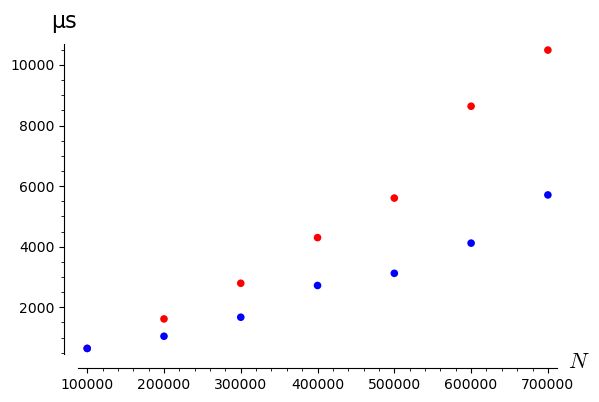In :
# Note: the linear complexity of the computation comes from
# the coefficient growth of the polynomial powers. Working modulo
# a prime results in a *logarthmic* complexity. For instance, here
# is the timing plot computing terms modulo 3.
R3.<x> = GF(3)['x']
J3 = R3.ideal(x^2-x-1)
K3 = R3.quo(J3)
xb2 = K3(x)
timesQ3 = [timeit('bin_pow(xb2,10^' + str(k) + ')') for k in range(10)]
point([(10^k,format_time(timesQ3[k])) for k in range(10)], scale="loglog", size=30)

Out: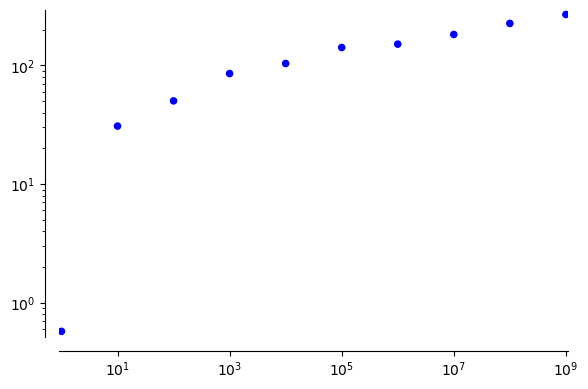## 3. Limit Theorems for the Euclidean GCD Algorithm Over Finite Fields¶

Let $e_{k,n}$ denote the number of pairs of polynomials $(f,g)$ over the finite field of prime order $p$ such that $f$ is monic, $n = \deg f > \deg g$, and Euclid's gcd algorithm applied to $(f,g)$ performs $k$ divisions. The terms $e_{k,n}$ approach a normal distribution as $n\rightarrow\infty$. Below we randomly generate polynomial pairs in finite fields, count the number of divisions performed by the Euclidean algorithm, and compare the experimental results to the limiting distribution.

#### Code to Plot the Number of Divisions on Random Polynomial Pairs vs Limit Distribution¶

In :
# Define the polynomial ring GF(p)[x]
# CHANGE THE PRIME HERE IF YOU WANT TO PLAY AROUND
p = 3
R = PolynomialRing(GF(p),'x')
S = Integers(p)
x = R.gen()

# EASteps(A,B,0) returns the number of steps the Euclidean algorithm
# takes for the polynomials A and B
def EASteps(A,B,steps):
if B == 0:
return steps
(q,r) = A.quo_rem(B)
return EASteps(B,r,steps+1)

# Generate uniformly random polynomial of degree at most D
def rpol(D):
return add([S.random_element() * x^k for k in range(D+1)])

# Returns the number of steps the Euclidean algorithm takes for a
# random pair of polynomials with the larger one monic of degree D
def randomEASteps(D):
f = x^D + rpol(D-1)
g = rpol(D-1)
return EASteps(f,g,0)

# Define limiting density
var('s n P')
density = sqrt(2)*exp(-n*(s/n-1+1/P)^2*P^2/(2*(P-1)))/(2*sqrt(pi*n)*sqrt((P-1)/P^2))
show("The limiting density for the Euclidean Algorithm on polynomial pairs in \t", R," is \t", density.subs(P=p))

In :
# Set maximum degree (N) and number of pairs (K) of polynomials to generate
# With the default p = 3, N = 500, and K = 2000 this should take 5 - 10 seconds
# CHANGE N and K HERE IF YOU WANT TO PLAY AROUND
N = 500
K = 2000

# Generate list of division counts for K random polynomial pairs
lst = [randomEASteps(N) for k in range(K)]

# Plot the count of points
p1 = plot(point([(k,lst.count(k)) for k in range(N)],size=50,marker="x", color="black"))
# Plot the limiting density (scaled to count divisions instead of probabilities)
p2 = plot(K*density.subs(n=N,P=p),(min(lst),max(lst)), color="red", thickness=2)
# Superimpose the plots
pt = p1 + p2
# Set the axes of the plot
pt.axes_range(xmin=min(lst),xmax=max(lst),ymin=0,ymax=pt.get_minmax_data()['ymax'])
# Display the plot on screen
pt

Out: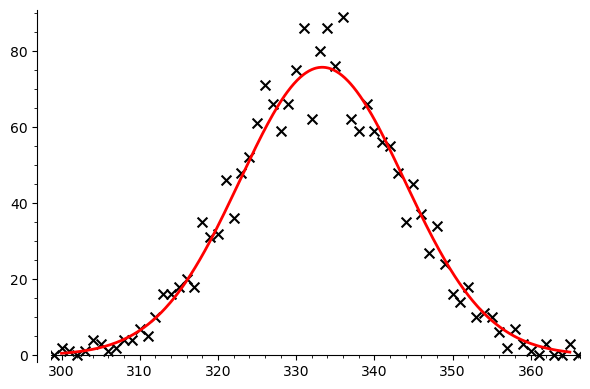#### Code to Animate Limit Theorems for the Euclidean GCD Algorithm Over Finite Fields¶

In :
# Returns the number of steps the Euclidean algorithm takes for a
# random pair of polynomials with the larger one monic of degree D
# *and* the polynomials used
def randomEAStepsfg(D):
f = x^D + rpol(D-1)
g = rpol(D-1)
return f,g,EASteps(f,g,0)

# Set maximum degree (N) and number of pairs (K) of polynomials to generate
N = 500
K = 2000

# Generate and record a sequence of K polynomial pairs with max degree N,
# together with the count of divisions performed by the Euclidean Algorithm
f_lst = []
g_lst = []
ct_lst = []
for step in range(K+1):
f, g, ct = randomEAStepsfg(N)
f_lst += [f]
g_lst += [g]
ct_lst += [ct]

# Generate a list of plots animating the running count of divisions
# compared to the expected count from the limit density
PT = []
ym = -1
for L in range(201):
step = 10*L
p1 = bar_chart([ct_lst[0:step+1].count(k) for k in range(N)], color='gray')
p2 = plot((step+1)*density.subs(n=N,P=p),(min(ct_lst),max(ct_lst)), color="red", thickness=2)
pt = p1 + p2
ym = max(ym,pt.get_minmax_data()['ymax'])
F = f_lst[step]
G = g_lst[step]
pt += text("$%s$" %("f(x) = " + latex(F.lt()) + "+ \\cdots +" + latex(F.constant_coefficient())),(0.03,1.05),axis_coords=True, horizontal_alignment="left", fontsize=14)
pt += text("$%s$"  %("g(x) = " + latex(G.lt()) + "+ \\cdots +" + latex(G.constant_coefficient())),(0.43,1.05),axis_coords=True, horizontal_alignment="left", fontsize=14)
pt += text("$%s$ runs"  %(step+1),(0.83,1.05),axis_coords=True, horizontal_alignment="left", fontsize=14)
pt.axes_range(xmin=min(ct_lst),xmax=max(ct_lst),ymin=0,ymax=ym)
PT += [pt]

# Generate an animation object from the list of plots
ani = animate(PT)

# Display the final frame of the animation
ani[-1]

Out: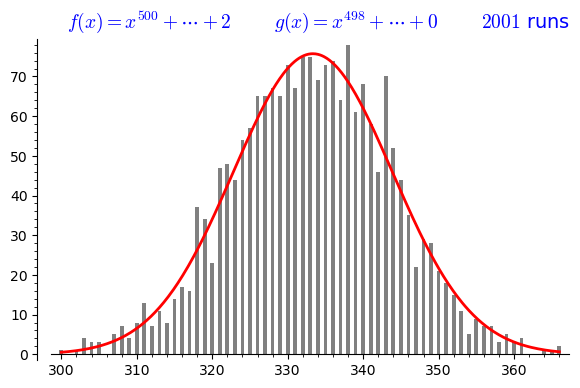In :
# Output and save the animation -- this *requires* extra software such as ImageMagick or ffmpeg
# Uncomment and run the following line to save the animation
# ani.save("EuclidAnimation.mp4")

In [ ]: